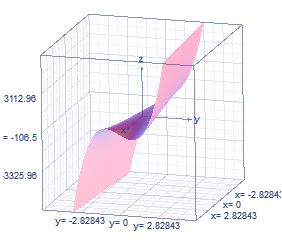# Find the local maximum and minimum values and saddle point(s) of the function. If you have...

## Question:

Find the local maximum and minimum values and saddle point(s) of the function. If you have three-dimensional graphing software, graph the function with a domain and viewpoint that reveal all the important aspects of the function. (Give your answers as a comma-separated list. If an answer does not exist, write DNE.)

{eq}f(x, y) = x^3 - 108xy + 216y^3 {/eq}

## Critical Points:

With the Hessian {eq}D(a,b) {/eq}we can know the type of critical point that has the function, if we equal the derivatives to zero we find the critical points.

{eq}D(a,b) > 0 \; \text{&} \; f_{xx} > 0 \; \Rightarrow \; \; \text{ f has a relative minimum point at (a,b, f(a,b))} \\ D(a,b) > 0 \; \text{&} \; f_{xx} < 0 \; \Rightarrow \; \; \text{ f has a relative maximum point at (a,b, f(a,b))} \\ D(a,b) < 0 \; \; \Rightarrow \; \; \text{then (a,b, f(a,b)) is a saddle point} \\ D(a,b) = 0 \Rightarrow \; \; \text{ the test is inconclusive} \\ {/eq}

We have the function

{eq}f(x, y) = x^3 - 108xy + 216y^3 \\ {/eq}

Graph the functionFirst partial derivatives:

{eq}f_x=3\,{x}^{2}-108\,y \\ {/eq}

{eq}f_y=648\,{y}^{2}-108\,x \\ {/eq}

Second partial derivatives:

{eq}f_{xx}= \\ f_{yy}= \\ f_{yx}= \\ {/eq}

Now,

{eq}f_x=0\,\, \Rightarrow \, 3\,{x}^{2}-108\,y =0\\ f_y=0\,\, \Rightarrow \, 648\,{y}^{2}-108\,x =0\\ {/eq}

The possible critical points are:

{eq}(0,0)\\ (6, 1) \\ {/eq}

Second derivative test for:

Point: {eq}(0,0) \\ {/eq}

{eq}f_{xx} (0,0)= 0\\ f_{yy} (0,0)= 0\\ f_{xy} (0,0)=-108 \\ {/eq}

Hessian is given by:

{eq}\displaystyle D(a,b)= f_{xx}(a,b).f_{yy}(a,b)-[ f_{xy} (a,b) ]^{2} \\ {/eq}

So, Hessian for {eq}(0,0) {/eq} is:

{eq}\displaystyle D(0,0)= f_{xx}(0,0).f_{yy}(0,0)-[ f_{xy} (0,0) ]^{2} \\ \displaystyle D(0,0)=0*0-[-108]^{2} \\ \displaystyle D(0,0)= -11664\\ {/eq}

{eq}\begin{array} \; \; \text{(a,b)} \; & { \ f_{xx} (a,b) } & { f_{yy} (a,b) } & f_{xy} (a,b) & D(a,b) & Conclusion & (a,b, f(a,b)) \\ \hline (0,0) & 0 & 0 & -108 & -11664 & Saddle \, point \; & (0,0, 0) \\ (6,1 ) & 36 & 1296 & -108 & 34992 & Relative \, minimum \; & ( 6,1 , -216) \\ \end{array} \\ {/eq}